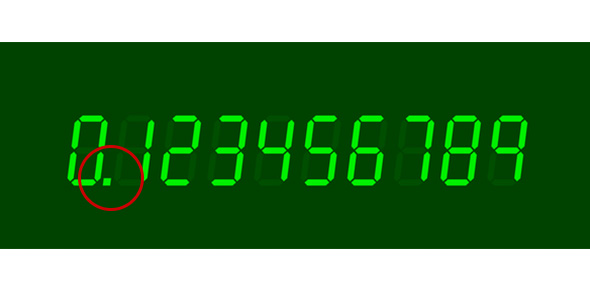# Converting Fractions To Decimal Numbers To Percents

10 Questions | Attempts: 775SettingsThis quiz is an assessment of being able to convert a fraction to a decimal number and converting decimal numbers to percents.

• 1.
Write this decimal number as a percent.0.56
• 2.
Write this decimal number as a percent.0.37
• 3.
Write this percent as a decimal number.50%
• 4.
Write this percent as a decimal number.3%
• 5.
What is this percent written in fraction and decimal form?5%
• A.

1/20 ; 0.5

• B.

1/10 ; 0.05

• C.

1/20 ; 0.05

• D.

1/15 ; 0.05

• 6.
What is this percent written in fraction and decimal form?99%
• A.

99/1,000 ; 0.99

• B.

99/100 ; 0.90

• C.

99/100 ; 0.099

• D.

99/100 ; 0.99

• 7.
Write this fraction as a decimal number.11/20
• 8.
Write this fraction as a decimal number.2/25
• 9.
What is this fraction written in decimal form and as a percent?9/20
• A.

0.37 ; 37%

• B.

0.45 ; 45%

• C.

0.045 ; 45%

• D.

0.40 ; 40%

• 10.
What is this fraction written in decimal form and as a percent?1/100
• A.

0.10 ; 1%

• B.

0.10 ; 10%

• C.

0.01 ; 10%

• D.

0.01 ; 1%

## Related TopicsBack to top
×

Wait!
Here's an interesting quiz for you.